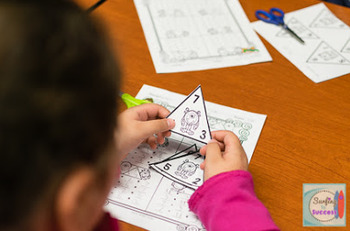# Fact Families: Add and Subtract within 18Subject
Resource Type
File Type

PDF

(18 MB|69 pages)
Standards
• Product Description
• Standards
Fact Family Addition and Subtraction to 18
Monster Theme

Focusing on Fact Families is a helpful strategy for learning the relationship between addition and subtraction. Some of my student seem to have an aversion to subtraction. When I show them that knowing how to add can help them subtract they see it as a short cut. Really, it helps develop basic algebraic understanding.

When I help older students, I find that students that used fact families to understand this relationship between addition and subtraction, have a better foundation for things like order of operations and algebra.

Included:

Triangle Flash Cards
Directions and Study Guide pages 3-4
Color Flashcards pages 5-12
Black and White Flashcards pages 13-20

Placemats for smartboard display, lamination or sheet protectors pages 21-24

Worksheet for use with all Flashcards sets page 25
Scaffolded version page 26

Art Extension Activities pages 27-31

Fact Family Dance Direction and Cards 32-44

Leveled Quizzes pages 45-52

Certificate page 53

**Update 3/3/16: I had a request to add zeros to the packet. I've added pages 60-69 which have color and black and white flashcards and leveled quizzes.**

Watch a video preview here.

Check out other
Fact Fluency resources here
.
Fluently add and subtract within 20 using mental strategies. By end of Grade 2, know from memory all sums of two one-digit numbers.
Add and subtract within 20, demonstrating fluency for addition and subtraction within 10. Use strategies such as counting on; making ten (e.g., 8 + 6 = 8 + 2 + 4 = 10 + 4 = 14); decomposing a number leading to a ten (e.g., 13 - 4 = 13 - 3 - 1 = 10 - 1 = 9); using the relationship between addition and subtraction (e.g., knowing that 8 + 4 = 12, one knows 12 - 8 = 4); and creating equivalent but easier or known sums (e.g., adding 6 + 7 by creating the known equivalent 6 + 6 + 1 = 12 + 1 = 13).
Apply properties of operations as strategies to add and subtract. If 8 + 3 = 11 is known, then 3 + 8 = 11 is also known. (Commutative property of addition.) To add 2 + 6 + 4, the second two numbers can be added to make a ten, so 2 + 6 + 4 = 2 + 10 = 12. (Associative property of addition.)
Total Pages
69 pages
Included
Teaching Duration
1 month
Report this Resource to TpT
Reported resources will be reviewed by our team. Report this resource to let us know if this resource violates TpT’s content guidelines.